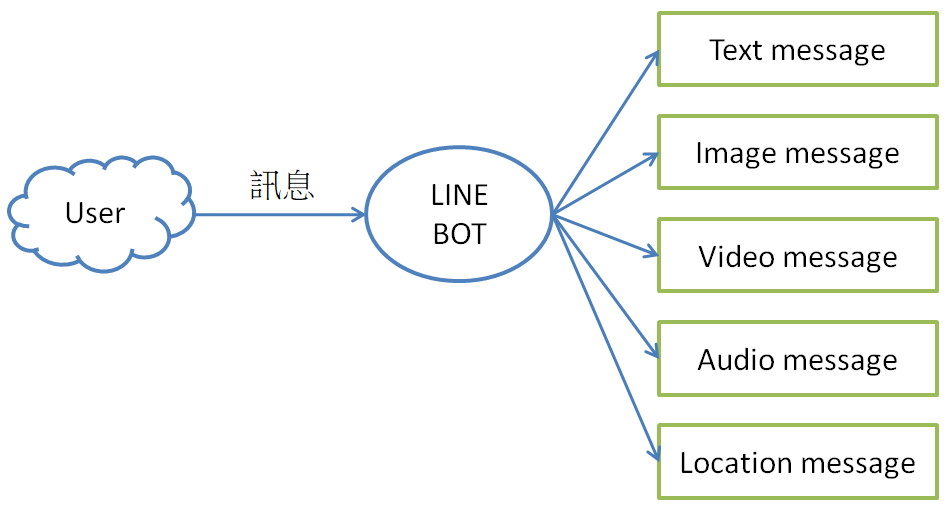#2
Software Development

## 工廠方法(Factory Method)

『工廠方法』顧名思義，這個設計模式是一個生產物件的工廠，使用者不須知道物件是如何生產的，只要指名要生產的物件名稱，工廠就會幫使用者生產所需的物件。例如，一個工廠有生產轎車(Car)、公車(Bus)、卡車(Truck)等車子，這些車種各自定義為一個類別如下：

``````class Car:
def __init__(self, maker='', num_of_doors=4,  fuel_type='oil'):
self.maker, self.num_of_doors,  self.fuel_type = maker, num_of_doors,  fuel_type

def __repr__(self):
return 'I\'m a car.'

class Bus:
def __init__(self, maker='', size=''):
self.maker, self.size = maker, size

def __repr__(self):
return 'I\'m a bus.'

class Truck:
def __init__(self, maker='', weight=10):
self.maker, self.weight = maker, weight

def __repr__(self):
return 'I\'m a truck.'
``````

``````def FactoryInterface(classname):
# 物件名稱與類別對照表
name_class_mapping = {'A':Car, 'B':Bus, 'C':Truck}

# 建立物件
return name_class_mapping[classname]()
``````

``````# 測試
obj1 = FactoryInterface('A')
print(obj1)

obj2 = FactoryInterface('B')
print(obj2)

obj3 = FactoryInterface('C')
print(obj3)
``````

I'm a car.
I'm a bus.
I'm a truck.

** 使用 eval()，甚至不需要 FactoryInterface 方法**，雖然方便，但不易偵錯，只有在執行時，才能發覺錯誤。

``````obj_name = 'Car'
obj = eval(obj_name + '()')
print(obj)
``````

## 產生有不同屬性的物件

1. 修改工廠方法：加一參數 arg，接收一個字典。
``````# 工廠方法
def FactoryInterface_2(classname, arg):
# 物件名稱與類別對照表
name_class_mapping = {'A':Car, 'B':Bus, 'C':Truck}

# 建立物件
return name_class_mapping[classname](**arg)
``````
1. 指定物件時，夾帶一個字典，指定物件各個參數及對應的值：
``````# 測試
arg = {'maker':'BMW', 'num_of_doors':4,  'fuel_type':'oil'}
obj1 = FactoryInterface_2('A', arg)
print(obj1)
print(obj1.maker)
print()

arg = {'maker':'Volvo', 'size':'large'}
obj2 = FactoryInterface_2('B', arg)
print(obj2)
print(obj2.size)
print()
``````

I'm a car.
BMW

I'm a bus.
large

## 接受多餘的參數

``````class Car:
def __init__(self, maker='', num_of_doors=4,  fuel_type='oil', **ignore):
self.maker, self.num_of_doors,  self.fuel_type = maker, num_of_doors,  fuel_type

def __repr__(self):
return 'I\'m a car.'

class Bus:
def __init__(self, maker='', size='', **ignore):
self.maker, self.size = maker, size

def __repr__(self):
return 'I\'m a bus.'

class Truck:
def __init__(self, maker='', weight=10, **ignore):
self.maker, self.weight = maker, weight

def __repr__(self):
return 'I\'m a truck.'
``````

``````# 多 size 參數
arg = {'maker':'BMW', 'num_of_doors':4,  'fuel_type':'oil', 'size':'large'}
obj1 = FactoryInterface_2('A', arg)
print(obj1)
print(obj1.maker)
print()

# 多 num_of_doors 參數
arg = {'maker':'Volvo', 'num_of_doors':4, 'size':'large'}
obj2 = FactoryInterface_2('B', arg)
print(obj2)
print(obj2.size)
print()
``````

I'm a car.
BMW

I'm a bus.
large

## 應用時機

『工廠方法』可以用在甚麼場合呢? 以筆者開發經驗，常發生在資料交換時，譬如 LINE BOT 訊息格式有很多種：

• Text message
• Sticker message
• Image message
• Video message
• Audio message
• Location message
• Imagemap message
• Template message
• Flex Message## 結語

1. 抽象工廠(Abstract Factory)
2. 物件創建者(Builder)